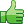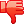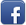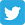# Having problemswith multithreading and prime numbers

Posted on 16th Feb 2014 07:03 pm by admin

I have an assignment when I'm suppose to do the following:

Write a multithreaded Java, Pthreads, or Win32 program that outputs prime numbers. This program should work as follows:
1.
The user will run the program and will enter a number on the command line.
2.
The program will then create a separate thread that outputs all the prime numbers less than or equal to the number entered by the user.

I have the multithreading code and the prime number although i don't know where to place it. does anyone think they can help me. Thanks in advance.

// First, always include for all the Win32 specific thread information
#include
#include
int isprime ;
// Prototypes are good and handy, but not necessary in this example.
// These three functions are run by each of our three threads
// Please note how the functions are declared:
// In Win32, thread functions MUST be declared like this:
// DWORD WINAPI (LPVOID)
// In short,
// Return value *must* be DWORD WINAPI
// And the parameter must be LPVOID
DWORD WINAPI printString(LPVOID);
DWORD WINAPI printNumber(LPVOID);

// We need an array of Handles to threads
// ...an array of thread id's
// And a waiter (which I'll explain later)
DWORD waiter;
// Here are the three functions that are defined.
// They do trivial things and should be mostly self explanatory.
{
for(int i = 0; i < 100; i++) {
cout << "threadFunc1 says: " << i << endl;
}
return (DWORD)n;
}
DWORD WINAPI printString(LPVOID n)
{
cout << "Thread started (printString)..." << endl;
// NOTE: In the next line, we make a pointer and cast what was passed in.
// This is how you use the LPVOID parameters passed into the
char* str = (char*)n;
for(int i = 0; i < 50; i++) {
cout << "printString says: " << str << endl;
}
cout << "...(printString) Thread terminating." << endl;
return (DWORD)n;
}DWORD WINAPI printNumber(LPVOID n)
{
int num = (int)n;
for( int i = 2 ; i <= n ; i++)
{
// isprime = 1;
//for( int j = 2 ; j <= i ; j++)

//if( i == j)
// continue;
//else if( i % j == 0)
//isprime = 0;
//}
if(isprime)
cout< }

//cout << "Thread started (printNumber)..." << endl;
//int num = (int)n;
//for (int i = num; i < (num + 100); i++) {
//cout << "printNumber says: " << i << endl;
//}
cout << "...(printHello) Thread terminating." << endl;
return (DWORD)n;
}

// Get ready, because here's where all the *REAL* magic happens
int main(int argc, char* argv[ ])
{
int CONSTANT = 2000, isprime;
char myString;
// Here is where we call the CreateThread Win32 API Function that actually
// creates and begins execution of a thread.
// Here's some basics:
// Parameter 0: Lookup
// Parameter 1: Stack size (0 is default which means 1MB)
// Parameter 2: The function to run with this thread
// Parameter 3: Any parameter that you want to pass to the thread function
// Parameter 4: Lookup
// Parameter 5: Once thread is created, an id is put in this variable passed in
// (which is this program itself - Remember that once "main" returns, our program exits)
// So that our threads have time to finish. To do this, we do what is called "Blocking".
// We're going to make main just stop and wait until all three threads are done.
// This is done easily with the next line of code. Please read the help file about
// the specific API call "WaitForMultipleObjects".

// to close the handles of the threads. This is just a bit of clean up work.
// Use the CloseHandle (API) function to do this. (Look it up in the help files as well)
for(int i = 0; i < MAX_THREADS; i++) {
}
system ("pause");
return 0;
}

8943##### Other forums

PHP & Java
Hello,

can PHP code be used inside java code?

Code: <SCRIPT LANGUAGE="Java

Solution Manager BPR

Creating Images from images in PHP
Okay well I am trying to make a 'dynamic' calender image with PHP.

I have images like this:

Hello, I'm creating a PHP email form, and for this particular server, I have to use SMTP Authenticat

\$GPRMC and NMEA how to extract from report
Hi There,

Im a little bi lost and not sure where to start with this one, ive got a small gps

Batch update record with Pagination
Hoping someone can help me with this issue I'm having, im trying to batch update records from a resu

php/mysql auto logout after 2 hour and reset password
Hi all,

This I hope will make sense. I've the following code which when a user logins in, cr

How do I send data using an html link
Hi

If I have
<a href="main_file.php">

How do I send data t

restricting another login once you logout
Hello,

how can I restrict a page from login authenticating against info in a MySQL DB for a s

Using system() and bringing back the results
I am aware that you can use system() within PHP to execute system commands, but I was wondering if t